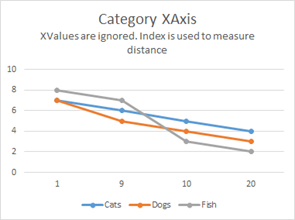iOS & macOS Charting Documentation - SciChart iOS & macOS Charts SDK v4.x

# Axis APIs - Value Axis vs. Category Axis

SciChart 2D iOS Features several axis types which could be Value or Category. All inherit from `SCIAxisBase`. So what’s the difference between Value and Category Axes? An explanation is given below.

Given the data:

Age (X-Axis) Cats Dogs Fish
1 7 7 8
9 6 5 7
10 5 4 3
20 4 3 2

A Value X-Axis and Category X-Axis would display the data differently:

Category X-Axis Value X-AxisThe fundamental difference is a Value-Axis uses the X-Value to compute distance on the XAxis, whereas a Category-Axis uses the X-Index. Value-Axis are primarily used in scientific software where Category–Axis are typically used in financial software (e.g. stock charts).

SciChart features both Value-Axis and Category-Axis types.

Axis Type Value or Category Axis
SCINumericAxis Value Axis
SCILogarithmicNumericAxis Value Axis
SCIDateAxis Value Axis
SCICategoryDateAxis Category Axis